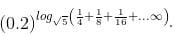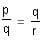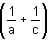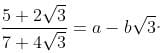CAT  >  Practice Questions Level 1: Exponents & Logarithm

# Practice Questions Level 1: Exponents & Logarithm - Notes | Study Level-wise Practice Questions for CAT Preparation - CAT

 1 Crore+ students have signed up on EduRev. Have you?

This EduRev document offers 10 Multiple Choice Questions (MCQs) from the topic Exponents & Logarithm (Level - 1). These questions are of Level - 1 difficulty and will assist you in the preparation of CAT & other MBA exams. You can practice/attempt these CAT Multiple Choice Questions (MCQs) and check the explanations for a better understanding of the topic.

Question for Practice Questions Level 1: Exponents & Logarithm
Try yourself:If log 3 = 0.47712, what will be the number of digits in 364?

Question for Practice Questions Level 1: Exponents & Logarithm
Try yourself:Solve the following equation for x:
log10 x - log10 √x = = 2 logx 10

Question for Practice Questions Level 1: Exponents & Logarithm
Try yourself:Find the value ofQuestion for Practice Questions Level 1: Exponents & Logarithm
Try yourself:If pa = qb = rc and, thenb =

Question for Practice Questions Level 1: Exponents & Logarithm
Try yourself:If 3x = 4y = 12z, find the value of z.

Question for Practice Questions Level 1: Exponents & Logarithm
Try yourself:The value of log4 3 × log5 4 x … x log9 8 × log3 9 is _______.

Question for Practice Questions Level 1: Exponents & Logarithm
Try yourself:Find a and b, ifQuestion for Practice Questions Level 1: Exponents & Logarithm
Try yourself:If the values of log10 2, log10 3 and log10 7 are known, which one of the following cannot be evaluated?

Question for Practice Questions Level 1: Exponents & Logarithm
Try yourself:The possible values of x for the equation log2 x2 + logx 2 = 3 are

Question for Practice Questions Level 1: Exponents & Logarithm
Try yourself:A sequence is given by log7 2, log7 4, log7 16, log7 256, ........... The common ratio of this geometric progression is

The document Practice Questions Level 1: Exponents & Logarithm - Notes | Study Level-wise Practice Questions for CAT Preparation - CAT is a part of the CAT Course Level-wise Practice Questions for CAT Preparation.
All you need of CAT at this link: CAT

## Level-wise Practice Questions for CAT Preparation

277 docs
 Use Code STAYHOME200 and get INR 200 additional OFF

## Level-wise Practice Questions for CAT Preparation

277 docs

### How to Prepare for CAT

Read our guide to prepare for CAT which is created by Toppers & the best Teachers

Track your progress, build streaks, highlight & save important lessons and more!

,

,

,

,

,

,

,

,

,

,

,

,

,

,

,

,

,

,

,

,

,

;